NCERT Class 12 Chemistry Solutions for Chapter 8  d-and f-Block Elements provides solutions to the questions provided in the textbook. These solutions are provided by the subject experts and are helpful for the students appearing for boards or competitive exams. The students can refer to these and enhance their conceptual knowledge.

NCERT Solutions are provided in the CBSE, MP, UP, and Gujarat boards. The students appearing for these boards can practice through the NCERT Solutions to score well in the examination.

 Board CBSE Textbook NCERT Class Class 12 Subject Chemistry Chapter Chapter 8 Chapter Name d-and f-Block Elements Number of Questions Solved 48 Category NCERT Solutions

## NCERT Solutions for Class 12 Chemistry Chapter 8 d-and f-Block Elements

The chapter d and f block elements is very important from the examination perspective. It explains the elements in the groups 3-12. A detailed understanding of this chapter will help the students to differentiate between the characteristics of d and f block elements. It also explains a comparative account of lanthanoids and actinoids.

The NCERT Solutions for Class 12 Chemistry Chapter 8 provides in-depth details of d and f blocks elements. The students are advised to refer to these solutions for better results.

NCERT IN-TEXT QUESTIONS

Question 1.
Silver has completely filled d-orbitals (4d10) in its ground state. How can you say that it is a transition metal ?
Silver (Z = 47) belongs to group 11 of (7-block (Cu, Ag, Au) and its outer electronic configuration is 4d105s1. It shows + 1 oxidation state (4d10 configuration) in silver halides (e.g. AgCl). However, it can also exhibit + 2 oxidation state (4d9 configuration) in compounds like AgF2 and AgO. Due to the presence of half filled d-orbital, silver is a transition metal.

Question 2.
In the series Sc (Z = 21) to Zn (Z = 30), the enthalpy of atomisation of zinc is the lowest, i.e., 126 kJ mol-1. Why?
In 3d series from Sc to Zn, all elements have one or more unpaired e-1 s except Zn which has no unpaired electron as its outer EC is 3d104s2. Hence, the intermetallic bonding is weakest in zinc. Therefore, enthalpy of atomisation is lowest.

Question 3.
Which of the 3d series of transitional metals exhibits largest oxidation states and why?
Mn (Z = 25) with electronic configuration [Ar]3d54s2 shows maximum oxidation state (+ 7) in its compounds since it has the maximum number of unpaired five i.e., seven. It shows largest variable oxidation state from + 2 to + 7 ( + 2, + 3, + 4, + 5, + 6, + 7) in its compounds.

Question 4.
The E°(M2+/M) value for copper is positive (+ 0·34 V). What is possibly the reason for this ? (C.B.S.E. Outside Delhi 2012, Sample Paper 2012)
E°(M2+/M) for any metal is based upon three factors which have been discussed in the text part.
M(s) + ∆aH → M(g) ; (∆aH = Enthalpy of atomisation)
M(g) + ∆fH → M2+(g) ; (∆fH = Ionisation enthalpy)
M2+(g) + aq → M2+(aq); (∆hydH = Hydration enthalpy)
Copper has very high enthalpy of atomisation (energy required) and low enthalpy of hydration (energy released). In nut shell, the ∆fH i.e. ionisation enthalpy needed is not compensated by the energy released. Therefore E°(Cu2+/Cu) is positive.

Question 5.
How would you account for the irregular variation in ionisation enthalpies (first and second) in first series of transition elements ?
The ionisation enthalpies of the transition metals are higher than those of s-block elements and less than the elements of p-block. Thus, these are less electropositive than the elements of s-block and at the same time more electropositive than the elements belonging to p-block present in the same period. In a transition series, the ionisation enthalpies increase from left to right. However, the gaps in the values of the two successive elements are not regular.

Question 6.
Why is the highest oxidation state of a metal exhibited in its oxide or fluoride only?
Oxygen and fluorine have small size and high electronegativity. Hence, they can oxidise the metal to the highest O.S.

Question 7.
Which is a stronger reducing agent Cr2+ or Fe2+ and why ? (SamplePaper 2011, C.B.S.E. Outside Delhi 2010, 2014)
Cr2+ is a stronger reducing agent than Fe2+. This is quite evident from the E° values ;
E°Cr3+/Cr2+ = – 0-41 V and E°Fe3+/Fe2+ = 0-77 V.
Reason : d4 → d3 occurs when Cr2+ changes to Cr3+ ion while d6 → d5 takes place when Fe2+ gets converted to Fe3+ ion.Now, d4 → d3 transition is easier as compared to d6 → d5 transition because in the second case, an electron is removed from a paired orbital which is rather difficult. Therefore, Cr2+ is a stronger reducing agent than Fe2+.

Question 8.
Calculate the spin magnetic moment of M2+(aq) ion (Z = 27).
Electronic configuration of element M(Z = 27) : [Ar] 3d74s2
Electronic configuration M2+ (aq) ion : 3d7 or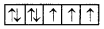Magnetic moment of M2+ (aq) ion with n = 3 ; $$\mu =\sqrt { n\left( n+2 \right) }$$
$$=\sqrt { 3\left( 3+2 \right) } =\sqrt { 15 } =3\cdot 87BM.$$

Question 9.
Explain why Cu+ ion is not stable in an aqueous solution. (C.B.S.E. Delhi 2011)
In aqueous solution Cu+ (aq) undergoes disproportionation to form Cu2+ (aq) ion and Cu.
2Cu+(aq) → Cu2+(aq) + Cu(s)
The higher stability of Cu2+ (aq) in an aqueous solution may be attributed to its greater negative ∆hydH than that of Cu+ (aq). It compensates for the second ionisation enthalpy of Cu involved in the formation of Cl2+ ion. Thus, Cu+ (aq) ion changes to Cu2+ (aq) ion which is more stable.

Question 10.
Actinoid contraction is greater from element to element than lanthanoid contraction. Why?
This is due to poor shielding by 5f-electrons in the actinoids than that by 4f e-1s in lanthanoids.

NCERT EXERCISE

Question 1.
Write down the electronic configuration of :
(a) Cr3+
(b) Cu+
(c) Co2+
(d) Mn2+
(e) Pm3+
(f) Ce4+
(g) Lu2+
(h) Th4+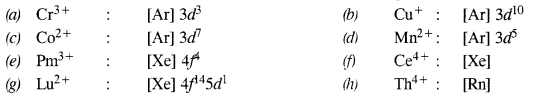Question 2.
Why are Mn2+ compounds more stable than Fe2+ towards oxidation to their+3 state?
A half-filled d orbital or a completely filled d orbital is more stable than any other state. Mn2+ already has a half-filled stable state hence would not undergo oxidation to form Mn+3. On the other hand, Fe2+ on oxidation to Fe3+ will have half-filled d orbitals which are more stable.

Question 2.
Why are Mn2+ compounds more stable than Fe2+ compounds towards oxidation to their +3 state?
Electronic configuration of Mn2+ is 3d5 while that of Fe2+ is 3d6. This shows that the Fe2+ ion has an urge to change to Fe3+ ion by losing an electron whereas the Mn2+ ion has no such tendency. Thus, the + 2 oxidation state of Mn is more stable as compared to the + 2 oxidation state of Fe.

Question 3.
Explain briefly how + 2 oxidation state becomes more and more stable in the first half of the first-row transition elements with increasing atomic number.
In all the elements listed, with the removal of valence 45 electrons (+2 oxidation state), the 3d-orbitals get gradually occupied. Since the number of empty d-orbitals decreases or the number of unpaired electrons in 3d orbitals increases, the stability of the cations (M2+) increases from Sc2+to Mn2+.

Question 4.
To what extent do the electronic configurations decide the stability of oxidation states in the first series of the transition elements? Illustrate your answer with an example.
In the transition series, the oxidation states which lead to exactly half-filled or completely filled d-orbitals are more stable. For example, the electronic configuration of Fe(Z = 26) is [Ar] 3d64s2. It shows various oxidation states but Fe (III) is most stable because it has the configuration [Ar]3d5.

Question 5.
What must be the stable oxidation state of the transition elements with the following electronic configuration in the ground states of their atoms: 3d3, 3d5, 3d8, 3d4?
The maximum oxidation states of reasonable stability in the transition metals of 3d series correspond to the sum of s and d-electrons upto Mn. However, after Mn there is an abrupt decrease in oxidation states. In the light of this, most stable oxidation states of the elements are:
3d3 : 3d3s2 (+ 5);3 : 3d54s1 (+ 6) and 3d54s2 (+ 7)
3d8 : 3d84s2 (+ 2); 3d4 : 3d44s2 or 3d54s1 (+6)

Question 6.
Name the oxometal anions in the first transition series of transition metals in which the metal exhibits an oxidation state equal to its group number.
chromate: CrO42-
permanganate: MnO4

Question 7.
What is lanthanoid contraction? What are the consequences of lanthanoid contraction? (C.B.S.E. Delhi 2013)
One common property associated with the elements in the periodic table is the variation in their atomic and ionic radii down the group and along a period. In general, these increase down the group due to the increase in the number of shells and decrease along a period considerably because of the increase in the magnitude of the effective nuclear charge.
Consequences of Lanthanoid Contraction
(a) Separation of Lanthanoids: Separation of lanthanoids is possible only due to lanthanoid contraction. All the lanthanoids have quite similar properties and due to this reason, they are difficult to separate. However, because of lanthanoid contraction, their properties (such as ionic size, ability to form complexes etc.,) vary slightly.

(b) Variation in basic strength of hydroxides: The basic strength of oxides and hydroxides decreases from La(OH)3 to Lu(OH)2. Due to lanthanoid contraction, size of M3+ ions decreases and thus there is a corresponding increase in the covalent character in M—OH bond. The acidic strength which involves the cleavage of the O—H bond follows the reverse trend i.e. it increases along the series.

(c) Similarly in the atomic sizes of the elements of the second and third transition series present in the same group: We know that the atomic sizes of the elements generally increase appreciably down a group. Similar trend is also expected in the elements present in the different groups of d-block.

(d) Variation in standard reduction potential: Due to lanthanoid contraction there is a small but steady increase in the standard reduction potential (E°) for the reduction process.
M3+ (aq) + 3e → M (aq)

(e) Variation in physical properties like melting point, boiling point, hardness etc: Various physical properties like m.pt., b.pt., hardness etc., increase with the increase in atomic number. This is because the attraction forces between the atoms increase as the size decreases.

Question 8.
What are the characteristics of transition elements and why are they called transition elements? Which of the d- block elements may not be regarded as the transition elements?
In the transition elements, d-orbitais are successively filled. The general electronic configuration of transition elements is (n – 1) d1-10 ns1-2. There are three transition series. The first transition series involves the filling of 3d-orbitais. It starts from scandium (Z = 21) and goes upto zinc (Z = 30).

The second transition series involves the filling of 4 d-orbitais and includes 10 elements from yttrium (Z = 39) to cadmium (Z = 48). The third transition series invokes the filling of 5d-orbitals. The first element of this series lanthanum (Z = 57). It is followed by fourteen elements called lanthanides which involve the filling of 4f-orbitais. The net nine elements from hafnium (Z = 72) to mercury (Z = 80) belong to the third transition series.

There is an incomplete fourth transition series. it involves the filling of 6d- subshell starting from actinium (Z = 89) followed by elements with atomic number 104 onwards.

Question 9.
In what way is the electronic configuration of the transition elements different from that of the non-transition elements?
Transition elements contain partially filled d-orbitals whereas non-transition elements have no d-orbitals or have completely filled d -orbitals.

Question 10.
What are the different oxidation states exhibited by lanthanoids?
+3 is the common oxidation state of the lanthanoids.
In addition to +3, oxidation states +2 and +4 are also exhibited by some of the lanthanoids.

Question 11.
Explain giving the reason:
(a) Transition metals and many of their compounds show paramagnetic behaviour. (H.P. Board 2014)
(b) The enthalpies of atomisation of transition metals are high. (Jharkhand Board 2010, C.B.S.E. Outside Delhi 2008, 2012, H.P. Board 2014)
(c) The transition metals generally form coloured compounds. (C.B.S.E. 2010. 2012)
(d) The transition metals and their compounds act as good catalysts. (C.B.S.E.Outside Delhi 2010) (C.B.S.E. Delhi 2008, Sample Paper 2010, H.P. Board 2017)
(i) Paramagnetic arises due to the presence of unpaired electrons, each such electron has a magnetic moment associated with it due to its spin angular momentum. Transition metals have in its ground state or ionized state has a number of unpaired d-electrons which gives them a paramagnetic behaviour.

(ii) Transition metals have very high interatomic metallic interaction due to the involvement of greater number of electrons from (n – l)d in addition to the ns electrons. The greater the number of valence electrons, the stronger is the resultant bonding due to the greater overlapping of half-filled orbitals. Hence, more amount of energy is required to break these metallic bonds. Thus enthalpy of atomisation of transition metal is very high.

(iii) Colour of transition metal – compounds is due to the excitation of an electron from a lower energy d-orbital to a higher energy d orbital. The energy of excitation corresponds to the frequency of light absorbed and the colour observed corresponds to the complementary colour of the light absorbed (whose frequency lies on the visible region). The frequency of the light absorbed depends on the nature of ligand. Transition metals form coloured compounds due to the presence of vacant d-orbitals for the d-d transition of e’ which causes the colour.

(iv) The catalytic activity of transition metals is ascribed to their ability to adopt multiple oxidation states and to form complexes. Catalyst at a solid surface involve the formation of bonds between reactant molecules and atoms of the surface of the catalyst this has the effect of increasing the concentration of the reactants at the catalyst surface and also weakening of the bonds in the reacting molecules (activation energy is lowered. Transition metals have d and s orbitals to from these bonds.

Question 12.
What are interstitial compounds? Why are such compounds well known for transition metals?
Interstitial compounds are the compounds formed as a result of the trapping of atoms of small elements like H, N, C, B etc. in the crystal lattices of certain metals. These are non-stoichiometric in nature and are neither ionic nor covalent. In fact, no proper bonds exist in the atoms of metals and non-metals involved in these compounds. Transition metals have a tendency to form such compounds. A few examples are: TiC,
Mn4N, Fe3H, VH0·56, Vse0·98 and Fe0·94O etc.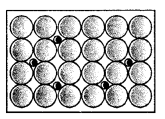1. These are generally non-stoichiometric in nature. Therefore, they cannot be represented by a definite structure or formula.
2. The compounds are neither covalent nor ionic and they don’t represent the normal oxidation states of the metals.
3. Since the strengths of the metallic bonds in these compounds increase due to greater electronic interactions, they show high melting points and high metallic conductivity. However, these compounds are chemically inert.
4. The conductivity of the metals remains unaffected in the corresponding interstitial compounds.

Question 13.
How is the variability in oxidation states of transition metals different from that of non-transition metals? Illustrate with examples.
The variability of oxidation states, characteristics of transition elements arises out of incomplete filling of d orbitals in such a way that their oxidation states differ from each other by unity This is in contrast with the variability of oxidation states of non-transition elements where oxidation states normally differ by a unit of two.
eg: Vanadium: V+2, V+3, V+4, V+5
Chromium : Cr+2, Cr+3, Cr+4, Cr+5, C+6
Nitrogen: +5, +3, +1, -1, -3.

Question 14.
Describe the preparation of potassium dichromate from chromite ore. What is the effect of increasing pH on a solution of potassium dichromate?
Preparation from chromite:
Potassium dichromate is generally prepared from chromite ore (FeCr2O4). It is in fact, a mixed oxide Fe0.Cr2O3 of iron and chrome also called ferrochrome or chrome iron.
(i) Conversion of chromite ore into sodium chromate: Chromite ore is fused with sodium hydroxide or sodium carbonate in the presence of air.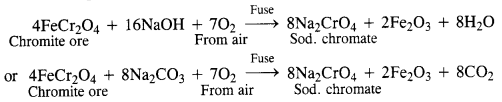(ii) Conversion of sodium chromate into sodium dichromate :
The fused mass obtained above is extracted with water. Sodium chromate which is soluble in water goes into the solution leaving behind the insoluble ferric oxide (Fe2O3). The yellow solution of sodium chromate obtained above is treated with concentrated H2SO4 to form sodium dichromate which has an orange colour.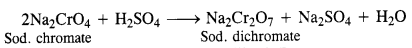(iii) Conversion of sodium dichromate into potassium dichromate:
Sodium dichromate is more soluble and less stable than potassium dichromate.Effect of increasing pH : The solution of potassium dichromate (K2Cr2O7) in water is orange in colour. On increasing the pH i.e. on adding the base, the potassium dichromate changes to potassium chromate (K2CrO4) which is yellow in colour. Thus, on increasing the pH, the colour of the solution changes from orange to yellow.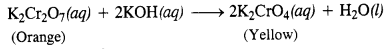Question 15.
Describe the oxidising action of potassium dichromate and write the ionic equations for its reaction with:
(a) iodide,
(b) iron (II) solution
(c) H2S.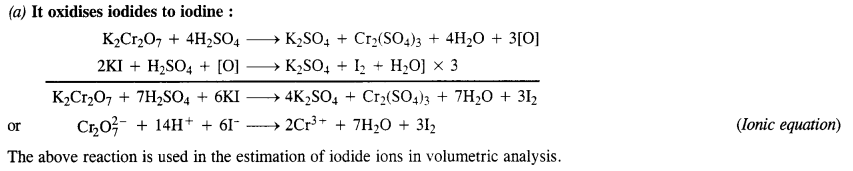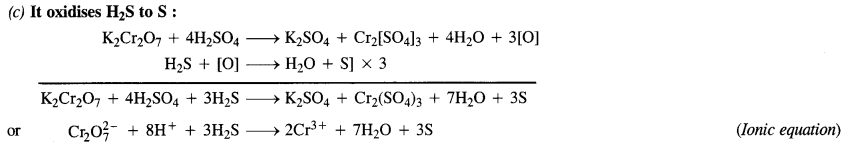Question 16.
Describe the preparation of potassium permanganate. How does acidified permanganate solution react with:
(a) iron (II) solution
(b) SO2
(c) oxalic acid?
Write the ionic equations for the reactions.
Potassium permanganate is prepared on a large scale from the mineral pyrolusite, MnO2.
2MnO2 + 4KOH + O2 → 2K2MnO4 + 2H2O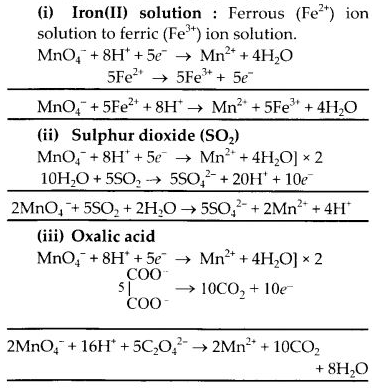Question 17.
For M2+/M and M3+/M2+ systems, the E° values of some metals are given :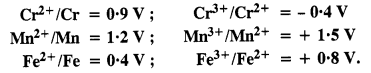Use this data to comment upon :
(a) The stability of Fe3+ in acid solution as compared to that of Cr3+ or Mn3+.
(b) The ease with which iron can be oxidised as compared to the similar process for either chromium or manganese metal.
As $${ E }_{ { Cr }^{ 3+ }/{ Cr }^{ 2+ } }^{ \circ }$$ is negative (-0·4 V), this means that Cr3+ ions in solution cannot be reduced to Cr2+ ions or Cr3+ ions are very stable. As farther comparison of E° values shows that Mn3+ ions can be reduced to Mn2+ ion more readily than Fe3+ ions. Thus, in the light of this, the order of relative stabilities of different ions is Mn3+ < Fe3+ < Cr3+.
(b) From the E° values, the order of oxidation of the metal to the divalent cation is Mn > Cr > Fe.

Question 18.
Predict which of the following will be coloured in an aqueous solution? Ti3+, V3+,Cu+, Sc3+, Mn2+, Fe3+ and Co2+ Give reasons for each.
Among the above mentioned, ions Ti3+, V3+ Mn2+, Fe3+, CO2+ will be coloured in its aqueous solution due to the ability of e’ to jump from a lower energy d orbital to a higher energy d orbital. In case of the ions Cu+, Sc3+ this d-d transition cannot take place either due to the absence of any e- in 3d orbital or due to complete filling of d orbital.

Question 19.
Compare the stability of the +2 oxidation state for the elements of the first transition series.
The common oxidation state of 3d series elements is + 2 which arises due to the participation of only 4s electrons. The tendency to show the highest oxidation state increases from Sc to Mn then decreases due to the pairing of electrons in 3d subshell. Thus in the series Sc(II) does not exist, Ti(II) is less stable than Ti(IV). At the other end of the series, the oxidation state of Zn is +2 only.

Question 20.
Compare the chemistry of actinoids with that of the lanthanoids with special reference to:
(i) electronic configuration
(ii) atomic and ionic sizes and
(iii) oxidation state
(iv) chemical reactivity.
(i) Electronic configuration:
The general electronic configuration for lanthanoids is [Xe] 0-14 5d 0-1 6s2 and that of actinoids is [Rn] 5f 0-14 6d0-1 7s2. Unlike 4f orbitals, 5 f orbitals are not deeply buried and participate in bonding to a greater extent.

(ii) Oxidation states:
The principal oxidation state of lanthanoids is (+3). However, sometimes we also encounter oxidation states of +2 and +4. This is because of extra stability of fully-filled and half-filled orbitals. Actinoids exhibit a greater range of oxidation states. This is because the 5f, 6d and 7s levels are of comparable energies. Again, (+3) is the principal oxidation state for actinoids. Actinoids such as lanthanoids have more compounds in +3 state than in +4 state.

(iii) Atomic and ionic size Similar to lanthanoids, actinoids also exhibit actinoid contraction overall decrease in atomic and ionic radii. The contraction is greater due to the poor shielding effect of 5f orbitals. Hence there is an unexpected in the atomic and ionic sizes of actinoids.

(iv) Chemical activity
In the lanthanide series, the earlier members of the series are more reactive. They have reactivity that is comparable to Ca. With an increase in the atomic number, the lanthanides start behaving similar to Al. Actinoids, on the other hand, are highly reactive metals, especially when they are finely divided. When they are added to boiling water, they give a mixture of oxide and hydride. Actinoids combine with most of the non-metals at moderate temperatures. Alkalies have no action on these actinoids. In the case of acids, they are slightly affected by nitric acid (because of the -formation of a protective oxide layer).

Question 21.
How would you account for the following :
(a) Of the d4 species, Cr2+ is strongly reducing while Mn3+ is strongly oxidising in nature.
(b) Cobalt (II) is stable in aqueous solution but in the presence of complexing reagents, it is easily oxidised.
(c) d1 configuration is very unstable in ions.
(a) E° value of Cr3+/Cr2+ is negative (-0·41 V) while that of Mn3+/Mn2+ is positive (+ 1·57 V). This means Cr2+ ions can lose electrons to form Cr3+ ions and act as a reducing agent while Mn3+ ions can accept electrons and can act as oxidising agent.
(b) Cobalt (II) is stable in aqueous solution but in the presence of complexing agent, it undergoes change in oxidation state from +2 to +3 and is easily oxidised.
(c) The ion with d1 configuration is expected to be extremely unstable and has a great urge to acquire d° configuration (very stable) by losing the only electron present in the d-subshell.

Question 22.
What is meant by ‘disproportionation’ ? Give two examples of disproportionation reaction in aqueous solution.
In a disproportionation reaction, an element undergoes an increase as well as decrease in its oxidation state forming
two different compounds. In other words, we can say that it can act both as reducing agent as well as oxidising agent.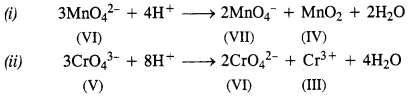Question 23.
Which metal in the first series of transition metals exhibits + 1 oxidation state most frequently and why?
Copper, because with +1 oxidation state an extra stable configuration, 3d10 results.

Question 24.
Calculate the number of unpaired electrons in the following gaseous ions :
Mn3+, Cr3+, V3+.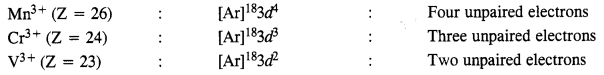Question 25.
Give examples and suggest reasons for the following features of the transition metal chemistry:
(i) The lowest oxide of transition metal is basic, the highest is amphoteric/acidic.
(ii) A transition metal exhibits the highest oxidation state in oxides and fluorides.
(iii) The highest oxidation state is exhibited in oxoanions of a metal.
(i) In case of a lower oxide of a transition metal, the metal atom has a low oxidation state. This means that some of the valence electrons of the metal atoms are not involved in bonding. As a result, it can donate electrons and behave as a base.

On the other hand, in the case of a higher oxide of a transition metal, the metal atom has a high oxidation state. This means that the valence electrons are involved in bonding. As a result, it can accept electrons and behave as an acid. For example, MnIIO is basic and MnVIIO is acidic.

(ii) Oxygen and fluorine act as strong oxidising agents because of their high electronegativities and small sizes. Hence, they bring out the highest oxidation states from the transition metals. In other words, a transition metal exhibits oxidation states in oxides and fluorides. For example, in OsF6 and V2O5, the oxidation states of Os and V are + 6 and respectively.

(iii) Oxygen is a strong oxidising agent due to its high electronegativity and small size so, oxoanions of metal have the highest oxidation state. For example, in MnO4, the oxidation state of Mn is +7.

Question 26.
Give the steps in the preparation of (C.B.S.E. Delhi 2009 comptt.)
(a) K2Cr2O7 from chromite ore
(b) KMnO4 from pyrolusite ore.
(a)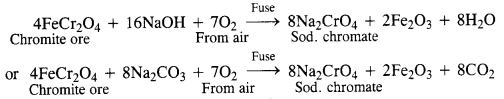(b)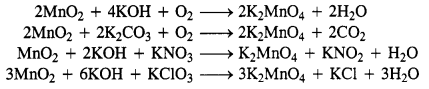Question 27.
What are alloys? Name an important alloy which contains some of the lanthanoid metals. Mention its uses.
An alloy is a homogeneous mixture of two or more metals or metals and non-metals. An important alloy that contains lanthanoid metal is mischmetal which contains 50% Cerium and 25 % Lanthanum, with small amounts of Nd (Neodymium) and Pr (Praseody-mium). It is used in Mg-based alloy to produce bullets, shells, and lighter flints.

Question 28.
What are inner transition elements? Decide which of the following atomic numbers belong to inner transition elements :
29, 59, 74, 95, 102, 104.
The inner transition elements also called/-block elements include the series of lanthanoids (Z = 58 to 71) and actinoids (Z = 90 to 103). This means that the elements with atomic numbers 59, 95, and 102 belong to inner transition elements.

Question 29.
The chemistry of actinoid elements is not so smooth as that of lanthanoids. Justify this statement by giving some examples of the oxidation states of these elements.
Lanthanoids show a limited number of oxidation states, such as +2, +3 and +4 (+3 is the principal oxidation state). This is because of large energy gap between 5d and 4f- subshells. On the other hand, actinoids also show a principal oxidation state of +3 but show a number of other oxidation state also. For example, Uranium (Z = 92) exhibits oxidation states of +3, +4, +5, +6 and Neptunium (Z = 94) shows oxidation states of +3, +4, +5, +6 and +7. This is because of small energy difference between 5f and 6d orbitals.

Question 30.
Which is the last element in the series of actinoids? Write the electronic configuration of the element. Comment upon its possible oxidation state.
Lawrencium (Lr = 103); [Rn] 5f146d17s2 oxidation state = +3.

Question 31.
Use Hund’s rule to derive the electronic configuration of Ce3+ ion and calculate its magnetic moment on the basis of the spin-spin formula.
Cerium electronic configuration = [Xe]4f1 5d1 16s2
Ce+3 ion = [Xe]4f1
i.e., one unpaired electron is present
Magnetic moment, µ = $$\sqrt{n(n+2)}$$ = $$\sqrt { 3 }$$ = 1.73BM.

Question 32.
Name the members of the lanthanoid series which exhibit+4oxidatk>nstatesandthosewhichexhibit +2 oxidation states. Try to correlate this type of behaviour with the electronic configurations of these elements.
+ 4 oxidation state in Ce (Z = 58), Pr (Z = 59), Tb (Z = 65).
+ 2 oxidation state in Nd (Z = 60), Sm(Z=62), Eu (Z = 63), Tm (Z=69), Yb (Z = 70).
+ 2 oxidation state is exhibited when the lanthanoid has the configuration 5cf 6s2 so that two electrons are-easily lost.
+ 4 oxidation state is exhibited by the elements which after losing four electrons acquire configuration 4f° or 4f1

Question 33.
Compare the chemistry of the actinoids with that of lanthanoids with reference to:
(i) electronic configuration
(ii) oxidation states and
(iii) chemical reactivity.
(i) Electronic configuration:
The general electronic configuration for lanthanoids is [Xe] 0-14 5d 0-1 6s2 and that of actinoids is [Rn] 5f 0-14 6d0-1 7s2. Unlike 4f orbitals, 5 f orbitals are not deeply buried and participate in bonding to a greater extent.

(ii) Oxidation states:
The principal oxidation states of lanthanoids is (+3). However, sometimes we also encounter oxidation states of +2 and +4. This is because of extra stability of fully-filled and half-filled orbitals. Actinoids exhibit a greater range of oxidation states. This is because the 5f, 6d and 7s levels are of comparable energies. Again, (+3) is the principal oxidation state for actinoids. Actinoids such as lanthanoids have more compounds in the +3 state than in the +4 state.

(iii) Atomic and ionic size Similar to lanthanoids, actinoids also exhibit actinoid contraction overall decrease in atomic and ionic radii. The contraction is greater due to the poor shielding effect of 5f orbitals. Hence there is an unexpected in the atomic and ionic sizes of actinoids.

(iv) Chemical reactivity
In the lanthanoid series, the earlier members of the series are more reactive. They have reactivity that is comparable to Ca. With an increase in the atomic number, the lanthanides start behaving similar to Al. Actinoids, on the other hand, are highly reactive metals, especially when they are finely divided. When they are added to boiling water, they give a mixture of oxide and hydride. Actinoids combine with most of the non-metals at moderate temperatures. Alkalies have no action on these actinoids. In the case of acids, they are slightly affected by nitric acid (because of the -formation of a protective oxide layer).

Question 34.
Write the electronic configuration of the elements with atomic numbers 61, 91, 101, 109.
Promethium or Pm (Z = 61) [Xe]544f55d06s2
Protactinium or Pa (Z = 91) [Rn] 4f26d17s2
Mendelevium or Md (Z = 101) [Rn] 5f16d07s2
Meitnerium or Mt (Z = 109) [Rn] 5f146d77s2

Question 35.
Compare the general characteristics of the first transition series of transition metals with those of the second and third transition series metals in the respective vertical columns. Give special emphasis on the following points :
(i) electronic configuration
(ii) oxidation states
(iii) ionisation enthalpies
(iv) atomic sizes.
(i) Electronic configuration. There are some exceptions in the electronic configurations in all the three series.(ii) Oxidation state. The elements belonging to the different series but present in the same group have similar electronic configurations and therefore, exhibit almost same variable oxidation states. In general, these are maximum in the middle of the series while minimum towards the end. Transition elements show variable oxidation states due to the participation of ns and (n – 1) d electrons in bonding because the energies of ns and (n – 1) d-subshells are quite close. The stability of a particular oxidation state depends upon the nature of the element with which the transition metal forms the compound.

(iii) Ionisation enthalpies. In general, the ionisation enthalpies in all three transition series increase from left to the right. However, the gaps in the two successive elements in a particular series are small and are also not regular. The first three ionisation enthalpies of the elements present in the first transition series are given in the text part. The ∆iH1 [ values of the elements belonging to the 5d series and higher as compared to those belonging to 3d and Ad series in the same group because of poor shielding by intervening 4f electrons present.

(iv) Atomic size. In all three transition series, the atomic, as well as ionic radii of the elements, increase from left to right. The values for 3d series are given in the text part. However, the increase in their values is not as much as expected since the shielding by (n – 1 )d electrons is not as much as expected. In a particular group, the atomic radius of the elements belonging to Ad series is more than the elements in the 3d series. However, the gaps in the elements belonging to Ad and 5d series are negligible on account of lanthanoid contraction which the elements of the 5d experience.

Question 36.
Write down the number of 3d electrons in each of the following ions :
Ti2+, V2+, Cr3+, Mn2+, Fe2+, Fe3+, Co2+, Ni2+ and Cu2+.
Indicate how would you expect the five 3d orbitals to be occupied for these hydrated ions (octahedral).
The number of 3d electrons in the ions are :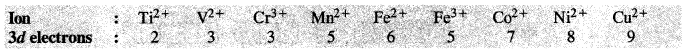For the explanation of the involvement of 3d orbitals in the hydrated ions (octahedral in nature) consult the next unit on coordination compounds.

Question 37.
Comment on the statement that elements of the first transition series possess many properties different from those of heavier transition elements.
The properties of the elements of the first transition series differ from those of the heavier transition elements in many ways.
(i) The atomic sizes of the elements of the first transition series are smaller than those of the heavier elements of the 2nd and 3rd transition series). However, the atomic sizes of the elements in the third transition series are virtually the same as those of the corresponding members in the second transition series this is due to lanthanoid configuration.

(ii) +2 and +3 oxidation states are more common for elements in the first transition series, while higher oxidation states are more common for the heavier elements.

(iii) The enthalpies of atomization of the elements in the first transition series are lower than those of the corresponding elements in the second and third transition series.

(iv) The melting and boiling points of the first transition series are lower than those of the heavier transition elements. This is because of the occurrence of strong metallic bonding (M – M bonding)

(v) The dements of the first transition series from low-spin or high-spin complexes depending upon the strength of the ligand field. However, the heavier transition elements form only low-spin complexes, irrespective of the strength of the ligand field.

Question 38.
What can be inferred from the magnetic moment values of the following complex species?The magnetic moment of a compound is given by the relation (µ) = $$\sqrt { n\left( n+2 \right) }$$ B.M, where n is the number of unpaired electrons.
For one unpaired electron (n = 1) ; µ = $$\sqrt { 1\left( 1+2 \right) } =\sqrt { 3 } =1\cdot 73\quad B.M.$$
For two unpaired electrons (n – 2) ; µ = $$\sqrt { 2\left( 2+2 \right) } =\sqrt { 8 } =2\cdot 83\quad B.M.$$
For three unpaired electrons (n = 3); µ = $$\sqrt { 3\left( 3+2 \right) } =\sqrt { 15 } =3\cdot 87\quad B.M.$$
For four unpaired electrons (n = 4) ; µ = $$\sqrt { 4\left( 4+2 \right) } =\sqrt { 24 } =4\cdot 9\quad B.M.$$
For five unpaired electrons (n = 5) ; µ = $$\sqrt { 5\left( 5+2 \right) } =\sqrt { 35 } =5\cdot 92\quad B.M.$$
*In the light of the above value, let us gather the desired information about the complex species that are mentioned
(i) K4[Mn(CN)6]
Oxidation state of Mn : [Mn(CN)6]4- , x + 6(-l) = -4 or x = -4 + 6 = + 2
The magnetic value of 1·73 B.M. indicates the presence of one unpaired electron in the complex. When six, CN ions (or ligands) approach Mn2+ ion, electrons in 3d orbitals pair up to make available six vacant orbitals involving d2sp3 hybridisation.The complex is octahedral and is paramagnetic due to one unpaired electron.
(ii) [Fe(H2O)6]2+
Oxidation state of Fe : [Fe(H2O)6]2+ ; r + 6 (0) = +2
The magnetic moment value of 5·3 B.M. indicates that there are four unpaired electrons in the complex. This means that the electrons in Fe2+ ion do not pair up when six H20 molecules (or ligands) approach it. Since the desired number of vacant orbitals (six) are available, die complex formed is sp3d2 hybridised.The complex is octahedral and is paramagnetic due to four unpaired electrons. It is also called outer orbital complex because 4d (n = 4) orbitals are involved.
(iii) K2[MnCl4]
Oxidation state of Mn : [MnCl4]2-, x + 4(-1) = -2 or x = -2 + 4= + 2
The magnetic moment value of 5·9 B.M. indicates that there are five unpaired electrons in the complex. This means that all the five 3d orbitals in Mn2+ ion are involved in the bond formation. The complex is sp3 hybridized in which one vacant 4s and three vacant 4p orbitals participate.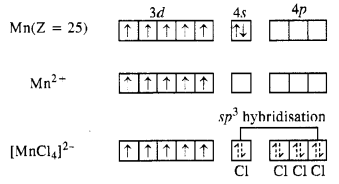The complex is therefore, tetrahedral in nature.

We hope the NCERT Solutions for Class 12 Chemistry Chapter 8 d-and f-Block Elements help you. If you have any query regarding NCERT Solutions for Class 12 Chemistry Chapter 8 d-and f-Block Elements, drop a comment below and we will get back to you at the earliest.